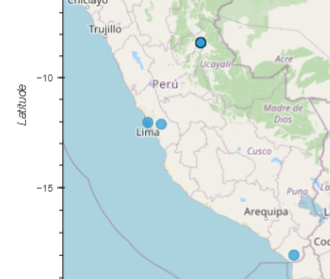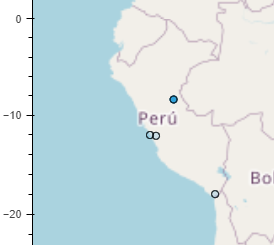# Overlaying second set of points based on selection stream from first set

Hello,

I am trying to plot a second set of points based on the selection from an initial set, but on the same plot.

The functionality I am aiming for is highlighting a group of unselected; related points (e.g. that the user might have missed when they made their selection).

I made a mock example with cities/countries

• dark circles borders should show up around all points from same country set that user selected from filled circles.
e.g. if the user selects a subset of the 4 cities in Peru, I want to show dark circles around the full set of all 4 cities.
``````import holoviews as hv
import geoviews as gv
import geoviews.tile_sources as gts
import pandas as pd
import geopandas
from holoviews import streams
import panel as pn

hv.extension('bokeh')

df = pd.DataFrame(
{'City': ['Buenos Aires', 'Brasilia', 'Santiago', 'Bogota', 'Caracas','Tacna','Pucallpa','Lima','Langa'],
'Country': ['Argentina', 'Brazil', 'Chile', 'Colombia', 'Venezuela','Peru','Peru','Peru','Peru'],
'Latitude': [-34.58, -15.78, -33.45, 4.60, 10.48, -18.006,-8.392,-12.046,-12.125],
'Longitude': [-58.66, -47.91, -70.66, -74.08, -66.86,-70.246,-74.582,-77.0427,-76.4211]})

gdf = geopandas.GeoDataFrame(df, geometry=geopandas.points_from_xy(df.Longitude, df.Latitude),crs="EPSG:3857")

gv_table = hv.Table(gdf)
tiles = gts.OSM.options(level='glyph')

point_size_slider = pn.widgets.IntSlider(name="Point Size",start=3,end=12,value=7)

@pn.depends(size=point_size_slider.param.value)
def base_points(size):
return gv.Points(gv_table,kdims=['Longitude','Latitude'],vdims=['City','Country']).opts(size=size,tools=['lasso_select'],nonselection_alpha=0.75)

test = hv.DynamicMap(base_points)

sel = streams.Selection1D(source=test)

@pn.depends(size=point_size_slider.param.value)
def highlight_missed(index,size):
'''
Add another layer of points (empty black circle outlines) for the selected points; but expand visible points to all that share country label
'''
if len(index)>0:
#TODO: is there a better way to slice a dynamic map and get the underlying data by index?
selected_countries = test.items().data.loc[index,'Country'].values[:]
return gv.Points(gdf[gdf['Country'].isin(selected_countries)],kdims=['Longitude','Latitude'],vdims=['City','Country']).opts(size=size,fill_color=None,line_width=1,line_color='black')
else:
return gv.Points(gdf,kdims=['Longitude','Latitude'],vdims=['City','Country']).opts(size=size+1,fill_color=None,line_width=1,line_color='black')

test2 = hv.DynamicMap(highlight_missed,streams=[sel])

plot = tiles*test*test2
layout = pn.Column(point_size_slider,plot.opts(width=600,height=600))
layout
``````

When I run this, it seems to only put the dark border around the points selected; not expanding the set to include the remaining points in the country:Success! I stumbled across this related one: Dynamically fetch other column value from selection1D stream

…and I think this revised section works:

``````point_size_slider = pn.widgets.IntSlider(name="Point Size",start=3,end=12,value=7)

@pn.depends(size=point_size_slider.param.value)
def base_points(size):
return gv.Points(gv_table,kdims=['Longitude','Latitude'],vdims=['City','Country']).opts(size=size,tools=['lasso_select'],nonselection_alpha=0.2)

test = hv.DynamicMap(base_points)

sel = streams.Selection1D(source=test)

def get_countries(table, index):
temp_ds = hv.Dataset(table.iloc[index]['Country'], 'Country')
#lst = (temp_ds[()].data['Country'].values[:])
return hv.Table(temp_ds[()].data['Country'])

def highlight_missed(table, index):
'''
Add another layer of points (empty black circle outlines) for the selected points; but expand visible points to all that share country label
'''
if len(index)>0:
selected_countries = list(hv.Dataset(table.iloc[index]['Country'], 'Country')[()].data['Country'])
return gv.Points(table.select(Country=selected_countries),kdims=['Longitude','Latitude'],vdims=['City','Country']).opts(size=7,nonselection_alpha=1.0,fill_color=None,line_width=1,line_color='black')
else:
return gv.Points(table,kdims=['Longitude','Latitude'],vdims=['City','Country']).opts(size=7,fill_color=None,line_width=1,line_color='black')

test2 = gv_table.apply(highlight_missed, streams=[sel])
id_dmap = gv_table.apply(get_countries, streams=[sel])

plot = tiles*test*test2
layout = pn.Row(pn.Column(point_size_slider,plot.opts(width=600,height=600)),id_dmap)
layout
``````

(also added the hv.table to help check things while troubleshooting)1 Like